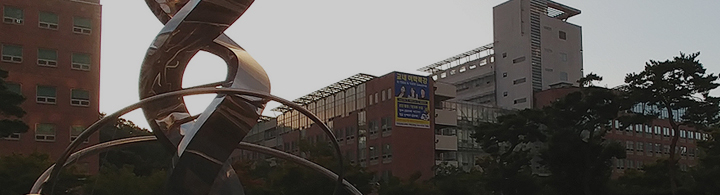ACADEMICS Global university challenghe and passion### Department of Applied Mathematics

“We foster competent mathematicians required by our society.”

Considering mathematical problems from the viewpoint of pure mathematics
Entering into statistics, finance, and computer system institutions

Applied mathematics is a field of mathematics that uses several theories of pure mathematics to solve various problems in natural and social science or undersatnds and solves the mathematical problems derived from these sciences in terms of pure mathematics.I ts theories have been actively applied to almost all fields such as engineering, computer, finance, insurance, information and communications, and marketing.
The department of applied mathematics aims to teach students basic theories of modern mathematics that form the foundation of natural science and several fields of applied mathematics and to foster talent having systematic knowledge with which they can actively cope with situations of modern industrial society.
In particular, the department has a large-scale, up-to-date computer room so that students can develop their mathematical problem-solving skills using computers, which are needed in the diversified fields of modern sciences, striving to foster useful mathematical talent by presenting education using statistics software or other math-related software.